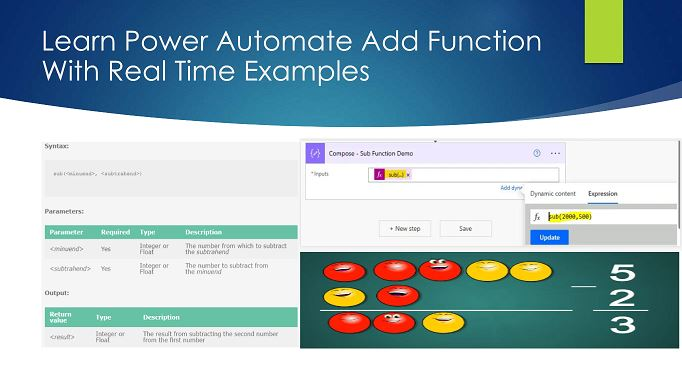# Power Automate Sub Function With Real Time ExamplesIn this “Power Automate Sub Function” article, we will learn about what a sub function is in Power Automate and how to use a sub function in Power Automate with real-time project-based examples.

Power Automate, a key component of Microsoft’s Power Platform, empowers users to automate tasks and processes seamlessly. Among its array of functions, the Sub function plays a crucial role in mathematical operations. In this article, we will dive into the capabilities of Power Automate Sub function by providing real-time examples to demonstrate how it can enhance productivity and simplify your work.

## What is the Sub Function in Power Automate?

The “Sub” function in Power Automate is designed for subtracting one number from another. Whether you need to calculate the difference between two numerical values, track expenses, or manage inventories, the “Sub” function proves its usefulness in a wide range of real-world scenarios. The sub function returns the result from subtracting the second number from the first number.

Syntax:

```sub(<minuend>, <subtrahend>)

```

Parameters:

 Parameter Required Type Description Yes Integer or Float The number from which to subtract the subtrahend Yes Integer or Float The number to subtract from the minuend

Output:

 Return value Type Description Integer or Float The result from subtracting the second number from the first number

## Power Automate Sub Function: Real-Time Examples

Let’s explore some practical examples of the Sub function in Power Automate:

### Example: Expense Tracking

Imagine you have a monthly expense tracking system in place, and you want to calculate the remaining budget after subtracting your expenses.

Example Demo:

```Set a variable: MonthlyBudget
MonthlyBudget = 2000 // Assuming your monthly budget is \$2000

Set a variable: Expense1
Expense1 = 500 // Expense for Rent

Set a variable: RemainingBudget
RemainingBudget = sub(MonthlyBudget, Expense1)```

Output:

The “Sub” function will subtract the expense from the monthly budget, and the variable RemainingBudget will store the remaining budget, which is \$1500 in this case.

### Example: Inventory Management

Suppose you manage an inventory system for your e-commerce store, and you need to keep track of the available stock after each sale.

Example Demo:

```Set a variable: CurrentStock
CurrentStock = 100 // Assuming you have 100 units in stock

Set a variable: SoldUnits
SoldUnits = 15 // Number of units sold

Set a variable: UpdatedStock
UpdatedStock = sub(CurrentStock, SoldUnits)```

Output:

The “Sub” function subtracts the number of units sold from the current stock, and the variable UpdatedStock will hold the updated stock quantity, which is 85 in this example.

### Example: Tracking Loan Payments

In a financial scenario, you might need to calculate the outstanding loan balance after a payment.

Example Demo:

```Set a variable: LoanAmount
LoanAmount = 10000 // Initial loan amount

Set a variable: MonthlyPayment
MonthlyPayment = 500 // Monthly installment

Set a variable: RemainingBalance
RemainingBalance = sub(LoanAmount, MonthlyPayment)```

Output:

The “Sub” function deducts the monthly payment from the initial loan amount, and the variable RemainingBalance will store the remaining loan balance, which is \$9,500 after the first payment.

### Example: Employee Leave Balances

For HR and leave management, you can use the “Sub” function to calculate leave balances after an employee takes a day off.

Example Demo:

```Set a variable: AnnualLeaveBalance
AnnualLeaveBalance = 20 // Total annual leave entitlement

Set a variable: LeaveTaken
LeaveTaken = 1 // Days of leave taken

Set a variable: RemainingLeave
RemainingLeave = sub(AnnualLeaveBalance, LeaveTaken)```

Output:

The “Sub” function deducts the days of leave taken from the annual leave entitlement, and the variable RemainingLeave will store the remaining leave balance, which is 19 days after one day is taken.

### Example: Working with substring function Power Automate

This is one of the most common scenarios for sub-functions. For example, if you are working on a substring function in Power Automate where you have extracted a certain index position of a text or character by using the IndexOf, lastIndexOf, or nthIndexOf function, then you want to start substring  in a certain position before the indexed position dynamically, then we can use the sub function to reduce the indexed position of that character or text. For details, refer to this article on its uses: Cancel All Flow Runs Using Power Automate More Than 20 Flows

## Benefits of Using the Sub Function in Power Automate

Accurate Calculations: The “Sub” function ensures precise numerical subtractions, minimizing the risk of errors.

• Time Savings: Automating subtraction tasks eliminates the need for manual calculations, saving time and effort.
• Real-time Updates: The function allows you to maintain real-time data, crucial for financial, inventory, and HR management.
• Error Reduction: Automation reduces human error in calculations, leading to more reliable results.

## Summary: Sub Function in Power Automate

Thus, in this article, we have learned about what a sub function is in Power Automate and how to work with a sub function in Power Automate in a real-time project-based scenario.

Power Automate Sub function is a powerful tool for performing subtraction operations within your workflows. As showcased in the real-time examples, it has diverse applications, from expense tracking to inventory management and loan payments. By automating subtraction tasks, you can save time, improve accuracy, and enhance your overall productivity. Start leveraging the “Sub” function today to streamline your work and simplify complex mathematical calculations.